• ## 极大值及小值定义

千次阅读 2018-03-07 22:09:56
驻点不一定是极致点。 驻点不可导点是可能极值点。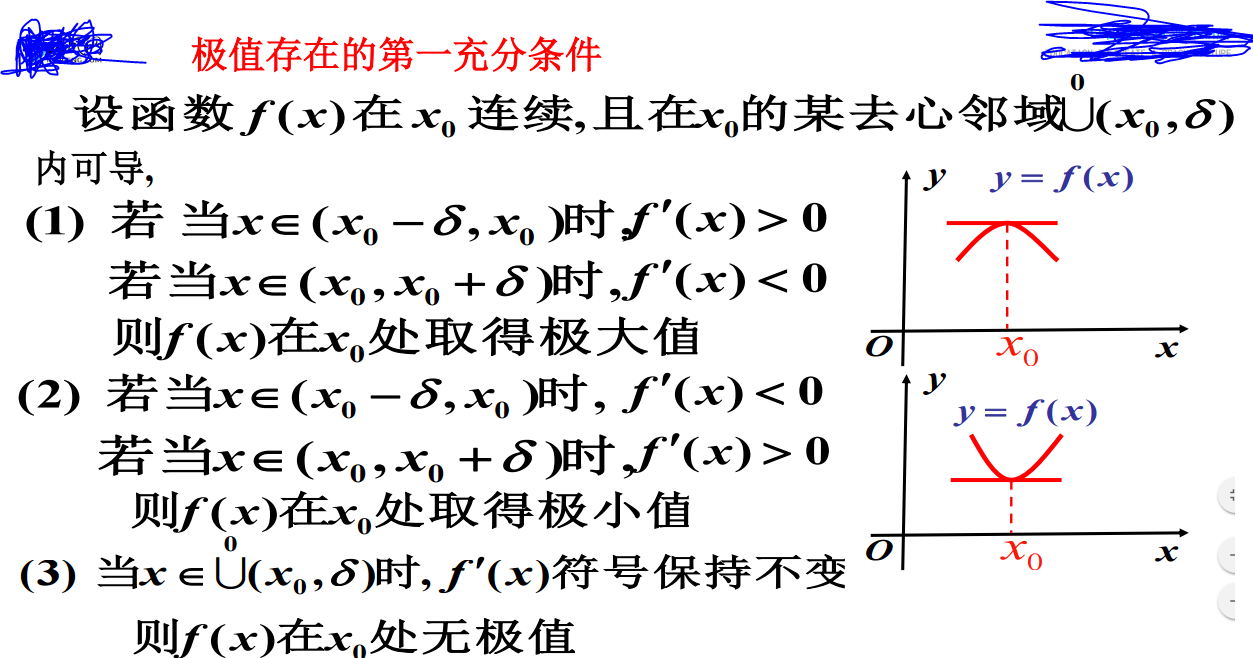驻点不一定是极致点。 驻点和不可导点是可能的极值点。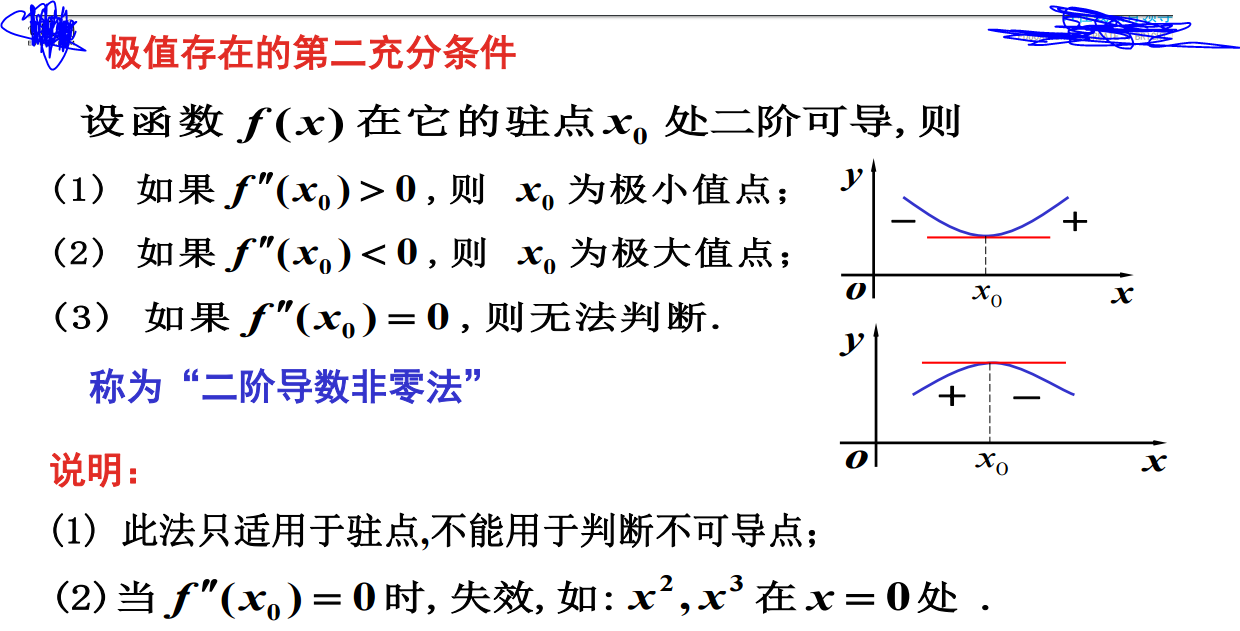展开全文• ## 极大极小值算法

万次阅读 2013-08-24 18:32:30
极小极大的定义 Minimax算法 又名极小极大算法，是一种找出失败的最大可能性中的最小值的算法（即最小化对手的最大得益）。通常以递归形式来实现。 Minimax算法常用于棋类等由两方较量的游戏程序。该算法是...
极小极大的定义 Minimax算法 又名极小化极大算法，是一种找出失败的最大可能性中的最小值的算法（即最小化对手的最大得益）。通常以递归形式来实现。

Minimax算法常用于棋类等由两方较量的游戏和程序。该算法是一个零总和算法，即一方要在可选的选项中选择将其优势最大化的选择，另一方则选择令对手优势最小化的一个，其输赢的总和为0（有点像能量守恒，就像本身两个玩家都有1点，最后输家要将他的1点给赢家，但整体上还是总共有2点）。很多棋类游戏可以采取此算法，例如tic-tac-toe。

关于极小极大，更多的信息可参考以下文章：
Minimax(wikipedia)

Understanding The MiniMax Algorithm

Minimax Explained

最小最大原理与搜索方法

以TIC-TAC-TOE为例
tic-tac-toe就是我们小时候玩的井字棋（我这边习惯叫“井字过三关”），不熟悉的可以参考wiki 。

一般X的玩家先下。设定X玩家的最大利益为正无穷（+∞），O玩家的最大利益为负无穷（-∞），这样我们称X玩家为MAX（因为他总是追求更大的值），成O玩家为MIN（她总是追求更小的值），各自都为争取自己的最大获益而努力。

现在，让我们站在MAX的立场来分析局势（这是必须的，应为你总不能两边倒吧，你喜欢的话也可以选择MIN）。由于MAX是先下的（习惯上X的玩家先下），于是构建出来的博弈树如下(前面两层)：MAX总是会选择MIN最大获利中的最小值（对MAX最有利），同样MIN也会一样，选择对自己最有利的（即MAX有可能获得的最大值）。有点难理解，其实就是自己得不到也不给你得到这样的意思啦，抢先把对对手有利的位置抢占了。你会看出，这是不断往下深钻的，直到最底层（即叶节点）你才能网上回溯，确定那个是对你最有利的。
具体过程会像是这么一个样子的：但实际情况下，完全遍历一颗博弈树是不现实的，因为层级的节点数是指数级递增的：
层数   节点数
0       1
1       9
2       72
3       504
4       3024
5       15120
6       60480
7       181440
8       362880

完全遍历会很耗时...一般情况下需要限制深钻的层数，在达到限定的层数时就返回一个估算值（通过一个启发式的函数对当前博弈位置进行估值），这样获得的值就不是精确的了（遍历的层数越深越精确，当然和估算函数也有一定关系），但该值依然是足够帮助我们做出决策的。于是，对耗时和精确度需要做一个权衡。一般我们限定其遍历的深度为6（目前多数的象棋游戏也是这么设定的）。

于是，我们站在MAX的角度，评估函数会是这样子的：

Java代码static   final   int   INFINITY = 100 ;   // 表示无穷的值
static   final   int   WIN = +INFINITY ;   // MAX的最大利益为正无穷
static   final   int   LOSE = -INFINITY ;   // MAX的最小得益（即MIN的最大得益）为负无穷
static   final   int   DOUBLE_LINK = INFINITY / 2 ;   // 如果同一行、列或对角上连续有两个，赛点
static   final   int   INPROGRESS = 1 ;   // 仍可继续下（没有胜出或和局）
static   final   int   DRAW = 0 ;   // 和局
static   final   int [][] WIN_STATUS =   {         {   0, 1, 2 },
{ 3, 4, 5 },
{ 6, 7, 8 },
{ 0, 3, 6 },
{ 1, 4, 7 },
{ 2, 5, 8 },
{ 0, 4, 8 },
{ 2, 4, 6   }
};   /**   * 估值函数，提供一个启发式的值，决定了游戏AI的高低   */  public   int   gameState( char []   board )   {          int   result = INPROGRESS;          boolean   isFull =   true ;                   // is game over?          for   ( int   pos = 0; pos < 9; pos++) {
char   chess = board[pos];                if   ( empty   == chess) {                     isFull =   false ;                      break ;               }         }          // is Max win/lose?          for   ( int [] status : WIN_STATUS) {                char   chess = board[status];                if   (chess ==   empty ) {                      break ;               }                int   i = 1;                for   (; i < status.length; i++) {                      if   (board[status[i]] != chess) {                            break ;                     }               }                if   (i == status.length) {                     result = chess ==   x   ? WIN : LOSE;                      break ;               }         }          if   (result != WIN & result != LOSE) {                if   (isFull) {                      // is draw                     result = DRAW;               }   else   {                      // check double link
// finds->'x', finds->'o'
int [] finds =   new   int ;
for   ( int [] status : WIN_STATUS) {                            char   chess =   empty ;                            boolean   hasEmpty =   false ;                            int   count = 0;                            for   ( int   i = 0; i < status.length; i++) {                                  if   (board[status[i]] ==   empty ) {                                       hasEmpty =   true ;                                 }   else   {                                        if   (chess ==   empty ) {                                             chess = board[status[i]];                                       }                                        if   (board[status[i]] == chess) {                                             count++;                                       }                                 }                           }                            if   (hasEmpty && count > 1) {                                  if   (chess ==   x ) {                                       finds++;                                 }   else   {                                       finds++;                                 }                           }                     }                      // check if two in one line
if   (finds > 0) {                           result = -DOUBLE_LINK;                     }   else   if   (finds > 0) {
result = DOUBLE_LINK;                     }               }         }          return   result;   }
static   final   int   INFINITY = 100 ;   // 表示无穷的值
static   final   int   WIN = +INFINITY ;   // MAX的最大利益为正无穷
static   final   int   LOSE = -INFINITY ;   // MAX的最小得益（即MIN的最大得益）为负无穷
static   final   int   DOUBLE_LINK = INFINITY / 2 ;   // 如果同一行、列或对角上连续有两个，赛点
static   final   int   INPROGRESS = 1 ;   // 仍可继续下（没有胜出或和局）
static   final   int   DRAW = 0 ;   // 和局
static   final   int [][] WIN_STATUS =   {
{   0, 1, 2 },
{ 3, 4, 5 },
{ 6, 7, 8 },
{ 0, 3, 6 },
{ 1, 4, 7 },
{ 2, 5, 8 },
{ 0, 4, 8 },
{ 2, 4, 6   }
};
/**
* 估值函数，提供一个启发式的值，决定了游戏AI的高低
*/
public   int   gameState( char []   board )   {
int   result = INPROGRESS;
boolean   isFull =   true ;

// is game over?
for   ( int   pos = 0; pos < 9; pos++) {
char   chess = board[pos];
if   ( empty   == chess) {
isFull =   false ;
break ;
}
}
// is Max win/lose?
for   ( int [] status : WIN_STATUS) {
char   chess = board[status];
if   (chess ==   empty ) {
break ;
}
int   i = 1;
for   (; i < status.length; i++) {
if   (board[status[i]] != chess) {
break ;
}
}
if   (i == status.length) {
result = chess ==   x   ? WIN : LOSE;
break ;
}
}
if   (result != WIN & result != LOSE) {
if   (isFull) {
// is draw
result = DRAW;
}   else   {
// check double link
// finds->'x', finds->'o'
int [] finds =   new   int ;
for   ( int [] status : WIN_STATUS) {
char   chess =   empty ;
boolean   hasEmpty =   false ;
int   count = 0;
for   ( int   i = 0; i < status.length; i++) {
if   (board[status[i]] ==   empty ) {
hasEmpty =   true ;
}   else   {
if   (chess ==   empty ) {
chess = board[status[i]];
}
if   (board[status[i]] == chess) {
count++;
}
}
}
if   (hasEmpty && count > 1) {
if   (chess ==   x ) {
finds++;
}   else   {
finds++;
}
}
}
// check if two in one line
if   (finds > 0) {
result = -DOUBLE_LINK;
}   else   if   (finds > 0) {
result = DOUBLE_LINK;
}
}
}
return   result;
} 

基于这些，一个限定层数的实现是这样的：

Java代码/**   * 以'x'的角度来考虑的极小极大算法   */  public   int   minimax( char [] board,   int   depth){          int [] bestMoves =   new   int ;          int   index = 0;                   int   bestValue = - INFINITY ;          for ( int   pos=0; pos<9; pos++){
if (board[pos]== empty ){                     board[pos] =   x ;                                           int   value = min(board, depth);                      if (value>bestValue){                           bestValue = value;                           index = 0;                           bestMoves[index] = pos;                     } else                     if (value==bestValue){                           index++;                           bestMoves[index] = pos;                     }                                          board[pos] =   empty ;               }                        }                   if (index>1){               index = ( new   Random (System. currentTimeMillis ()).nextInt()>>>1)%index;         }          return   bestMoves[index];            }   /**   * 对于'x'，估值越大对其越有利   */  public   int   max( char [] board,   int   depth){                   int   evalValue =   gameState (board);                   boolean   isGameOver = (evalValue== WIN   || evalValue== LOSE   || evalValue== DRAW );          if (depth==0 || isGameOver){                return   evalValue;         }                   int   bestValue = - INFINITY ;          for ( int   pos=0; pos<9; pos++){
if (board[pos]== empty ){                      // try                     board[pos] =   x ;                                           //   maximixing
bestValue = Math. max (bestValue, min(board, depth-1));                                           // reset                     board[pos] =   empty ;               }                        }                   return   evalValue;            }   /**   * 对于'o'，估值越小对其越有利   */  public   int   min( char [] board,   int   depth){                   int   evalValue =   gameState (board);                   boolean   isGameOver = (evalValue== WIN   || evalValue== LOSE   || evalValue== DRAW );          if (depth==0 || isGameOver){                return   evalValue;         }                   int   bestValue = + INFINITY ;          for ( int   pos=0; pos<9; pos++){
if (board[pos]== empty ){                      // try                     board[pos] =   o ;                                           //   minimixing
bestValue = Math.min(bestValue, max(board, depth-1));                                           // reset                     board[pos] =   empty ;               }                        }                   return   evalValue;            }
/**
* 以'x'的角度来考虑的极小极大算法
*/
public   int   minimax( char [] board,   int   depth){
int [] bestMoves =   new   int ;
int   index = 0;

int   bestValue = - INFINITY ;
for ( int   pos=0; pos<9; pos++){

if (board[pos]== empty ){
board[pos] =   x ;

int   value = min(board, depth);
if (value>bestValue){
bestValue = value;
index = 0;
bestMoves[index] = pos;
} else
if (value==bestValue){
index++;
bestMoves[index] = pos;
}

board[pos] =   empty ;
}

}

if (index>1){
index = ( new   Random (System. currentTimeMillis ()).nextInt()>>>1)%index;
}
return   bestMoves[index];

}
/**
* 对于'x'，估值越大对其越有利
*/
public   int   max( char [] board,   int   depth){

int   evalValue =   gameState (board);

boolean   isGameOver = (evalValue== WIN   || evalValue== LOSE   || evalValue== DRAW );
if (depth==0 || isGameOver){
return   evalValue;
}

int   bestValue = - INFINITY ;
for ( int   pos=0; pos<9; pos++){

if (board[pos]== empty ){
// try
board[pos] =   x ;

//   maximixing
bestValue = Math. max (bestValue, min(board, depth-1));

// reset
board[pos] =   empty ;
}

}

return   evalValue;

}
/**
* 对于'o'，估值越小对其越有利
*/
public   int   min( char [] board,   int   depth){

int   evalValue =   gameState (board);

boolean   isGameOver = (evalValue== WIN   || evalValue== LOSE   || evalValue== DRAW );
if (depth==0 || isGameOver){
return   evalValue;
}

int   bestValue = + INFINITY ;
for ( int   pos=0; pos<9; pos++){

if (board[pos]== empty ){
// try
board[pos] =   o ;

//   minimixing
bestValue = Math.min(bestValue, max(board, depth-1));

// reset
board[pos] =   empty ;
}

}

return   evalValue;

} 

Alpha-beta剪枝

另外，通过结合Alpha-beta剪枝能进一步优化效率。Alpha-beta剪枝顾名思义就是裁剪掉一些不必要的分支，以减少遍历的节点数。实际上是通过传递两个参数alpha和beta到递归的极小极大函数中，alpha表示了MAX的最坏情况，beta表示了MIN的最坏情况，因此他们的初始值为负无穷和正无穷。在递归的过程中，在轮到MAX的回合，如果极小极大的值比alpha大，则更新alpha；在MIN的回合中，如果极小极大值比beta小，则更新beta。当alpha和beta相交时（即alpha>=beta），这时该节点的所有子节点对于MAX和MIN双方都不会带来好的获益，所以可以忽略掉（裁剪掉）以该节点为父节点的整棵子树。

根据这一定义，可以很轻易地在上面程序的基础上进行改进：

Java代码/**   * 以'x'的角度来考虑的极小极大算法   */  public   int   minimax( char [] board,   int   depth){          int [] bestMoves =   new   int ;          int   index = 0;                   int   bestValue = - INFINITY ;          for ( int   pos=0; pos<9; pos++){
if (board[pos]== empty ){                     board[pos] =   x ;                                           int   value = min(board, depth, - INFINITY , + INFINITY );                      if (value>bestValue){                           bestValue = value;                           index = 0;                           bestMoves[index] = pos;                     } else                     if (value==bestValue){                           index++;                           bestMoves[index] = pos;                     }                                          board[pos] =   empty ;               }                        }                   if (index>1){               index = ( new   Random (System. currentTimeMillis ()).nextInt()>>>1)%index;         }          return   bestMoves[index];            }   /**   * 对于'x'，估值越大对其越有利   */  public   int   max( char [] board,   int   depth,   int   alpha,   int   beta){
int   evalValue =   gameState (board);                   boolean   isGameOver = (evalValue== WIN   || evalValue== LOSE   || evalValue== DRAW );          if (beta<=alpha){                return   evalValue;         }          if (depth==0 || isGameOver){                return   evalValue;         }                   int   bestValue = - INFINITY ;          for ( int   pos=0; pos<9; pos++){
if (board[pos]== empty ){                      // try                     board[pos] =   x ;                                           //   maximixing
bestValue = Math. max (bestValue, min(board, depth-1, Math. max (bestValue, alpha), beta));                                           // reset                     board[pos] =   empty ;               }                        }                   return   evalValue;            }   /**   * 对于'o'，估值越小对其越有利   */  public   int   min( char [] board,   int   depth,   int   alpha,   int   beta){
int   evalValue =   gameState (board);                   boolean   isGameOver = (evalValue== WIN   || evalValue== LOSE   || evalValue== DRAW );          if (alpha>=beta){                return   evalValue;         }          // try          if (depth==0 || isGameOver || alpha>=beta){                return   evalValue;         }                   int   bestValue = + INFINITY ;          for ( int   pos=0; pos<9; pos++){
if (board[pos]== empty ){                      // try                     board[pos] =   o ;                                           //   minimixing
bestValue = Math.min(bestValue, max(board, depth-1, alpha, Math.min(bestValue, beta)));                                           // reset                     board[pos] =   empty ;               }                        }                   return   evalValue;            }
/**
* 以'x'的角度来考虑的极小极大算法
*/
public   int   minimax( char [] board,   int   depth){
int [] bestMoves =   new   int ;
int   index = 0;

int   bestValue = - INFINITY ;
for ( int   pos=0; pos<9; pos++){

if (board[pos]== empty ){
board[pos] =   x ;

int   value = min(board, depth, - INFINITY , + INFINITY );
if (value>bestValue){
bestValue = value;
index = 0;
bestMoves[index] = pos;
} else
if (value==bestValue){
index++;
bestMoves[index] = pos;
}

board[pos] =   empty ;
}

}

if (index>1){
index = ( new   Random (System. currentTimeMillis ()).nextInt()>>>1)%index;
}
return   bestMoves[index];

}
/**
* 对于'x'，估值越大对其越有利
*/
public   int   max( char [] board,   int   depth,   int   alpha,   int   beta){

int   evalValue =   gameState (board);

boolean   isGameOver = (evalValue== WIN   || evalValue== LOSE   || evalValue== DRAW );
if (beta<=alpha){
return   evalValue;
}
if (depth==0 || isGameOver){
return   evalValue;
}

int   bestValue = - INFINITY ;
for ( int   pos=0; pos<9; pos++){

if (board[pos]== empty ){
// try
board[pos] =   x ;

//   maximixing
bestValue = Math. max (bestValue, min(board, depth-1, Math. max (bestValue, alpha), beta));

// reset
board[pos] =   empty ;
}

}

return   evalValue;

}
/**
* 对于'o'，估值越小对其越有利
*/
public   int   min( char [] board,   int   depth,   int   alpha,   int   beta){

int   evalValue =   gameState (board);

boolean   isGameOver = (evalValue== WIN   || evalValue== LOSE   || evalValue== DRAW );
if (alpha>=beta){
return   evalValue;
}
// try
if (depth==0 || isGameOver || alpha>=beta){
return   evalValue;
}

int   bestValue = + INFINITY ;
for ( int   pos=0; pos<9; pos++){

if (board[pos]== empty ){
// try
board[pos] =   o ;

//   minimixing
bestValue = Math.min(bestValue, max(board, depth-1, alpha, Math.min(bestValue, beta)));

// reset
board[pos] =   empty ;
}

}

return   evalValue;

} 
*这里对极小极大算法的实现只是其中一种可行性，实际上可能会看到很多种不同的实现方式，但道理是一样的。

使用开局库
同时，你会发现，这样做视乎还不够，特别在一开局。我们都知道，中心的位置是最好的，但是按照我们上面的算法，第一步确实随机的...这在深度受限制的情况下就更显得重要了。于是就引申出了开局库的概念，这是我在某个讲象棋AI的网上看到的，就是给初始的棋盘设定一些格局。

针对上面的例子，我们只要判断是否第一步棋，如果是则想办法让他选择中心的位置（4）。

在WIKI百科中找到一幅图也能作为TIC-TAC-TOE开局库的参考：于是，估算函数会变成这样的（当然也可以在别的地方修改，只要合理）：

Java代码//开局时，每个位置的估值   static   final   int []   INITIAL_POS_VALUE   = {         3, 2, 3,         2, 4, 2,         3, 2, 3  };   /**   * 估值函数，提供一个启发式的值，决定了游戏AI的高低   */  public   int   gameState ( char []   board ) {          int   result =   INPROGRESS ;          boolean   isFull =   true ;          int   sum = 0;          int   index = 0;          // is game over?          for ( int   pos=0; pos<9; pos++){
char   chess = board[pos];                if ( empty ==chess){                     isFull =   false ;               } else {                     sum += chess;                     index = pos;               }         }                   // 如果是初始状态，则使用开局库          boolean   isInitial = (sum== x ||sum== o );          if (isInitial){                return   (sum== x ?1:-1)*INITIAL_POS_VALUE[index];
}                   // is Max win/lose?          for ( int [] status :   WIN_STATUS ){                char   chess = board[status];                if (chess== empty ){                      break ;               }                int   i = 1;                for (; i<status.length; i++){                      if (board[status[i]]!=chess){                            break ;                     }               }                if (i==status.length){                     result = chess== x   ?   WIN   :   LOSE ;                      break ;               }         }                   if (result!= WIN   & result!= LOSE ){                               if (isFull){                      // is draw                     result =   DRAW ;               } else {                      // check double link
// finds->'x', finds->'o'
int [] finds =   new   int ;
for ( int [] status :   WIN_STATUS ){                            char   chess =   empty ;                            boolean   hasEmpty =   false ;                            int   count = 0;                            for ( int   i=0; i<status.length; i++){                                  if (board[status[i]]== empty ){                                       hasEmpty =   true ;                                 } else {                                        if (chess== empty ){                                             chess = board[status[i]];                                       }                                        if (board[status[i]]==chess){                                             count++;                                       }                                 }                           }                            if (hasEmpty && count>1){                                  if (chess== x ){                                       finds++;                                 } else {                                       finds++;                                 }                           }                     }                                           // check if two in one line
if (finds>0){                           result = - DOUBLE_LINK ;                     } else                     if (finds>0){                           result =   DOUBLE_LINK ;                     }                                    }                        }                   return   result;            }
转载地址：http://univasity.iteye.com/blog/1170216

展开全文• 局部极小值 一个给定的不包含相同元素的整数数组，每个，局部极小值的定义是一个值比左右相邻的（如果存在）都小的值，求它的一个局部最小值 分析： 局部最小值的存在性，全部数组的最小值显然是
数组简介

数组(array)

java : [], ArrayList
C++ : STL vector, []
C：  只有[]
理解：输入的数组通常理解为集合，我们自己可以排序，查找
注意

C++ STL中vector的一种实现
数组下标是一种特殊的hash…做计数
理解数组与map
给数组“顺序”

局部极小值

一个给定的不包含相同元素的整数数组，每个，局部极小值的定义是一个值比左右相邻的（如果存在）都小的值，求它的一个局部最小值

分析：

局部最小值的存在性，全部数组的最小值显然是一个解。 O(n)?
我们规定数组下标a[1…n]，并定义a = a[n + 1] = ∞, 我们有a < a, a[n] < a[n + 1]
结论： 子数组a[x…y] 若 a[x] < a[x – 1] , a[y] < a[y + 1]，则它包含一个局部极小值

二分法

mid = (x + y) / 2,二分，两个子数组a[x…mid], a[mid + 1…y]

若a[mid] < a[mid + 1], 则子数组a[x…mid]满足a[x] < a[x - 1], a[mid] < a[mid + 1]
反之a[mid] > a[mid + 1], 则子数组a[mid + 1…y]满足a[mid + 1] < a[mid], a[y] < a[y + 1]

复杂度 O(logn)

第一个缺失的正整数

给一个数组，找到从1开始第一个不在里面的正整数。
例如［3,4,-1,1]输出2。

分析: 数组下标从0开始
让a[i] == i + 1

每次循环
要么i ＋ 1
要么n – 1
要么有一个数
被放到正确的位置

代码实现

排序复杂度 O(logn)

class Solution{
public:
int firstMissingPositive(int A[],int n){
//(0...i) is (1...i)
for(int i=0;i<n;){
if(A[i]==i+1){
++i;
}else if((A[i]<=i)||(A[i]>n)||A(A[A[i]-1]==A[i])){
//小于等于i   大于n(最大值)    重复A=5 A[5-1]=A=A
A[i]=A[--n];//删除A[i],并把结尾的数交换到i的位置
}else{
// A A[5-1]=A=A
swap(A[i],A[A[i]-1]);
}
}
}
}

元素最大间距离

给定一个整数数组(n > 1)，求把这些整数表示在数轴上，相邻两个数差的最大值。

分析

显然排序是一个思想。有更好的方法么
最大值x, 最小值y, 如果x == y显然答案是0
把数放进(n + 1)个桶

每个桶大小是d = (x – y) / (n + 1) （浮点数）
每个桶区间是[y + i * d, y + (i + 1) * d) (i=0,1,…n)

注意是左闭右开的区间，最后一个桶是双闭区间
最小的数在0号桶里，最大的数在n号桶里
第一个桶非空，最后一个桶非空

中间有空桶，空桶左右两侧肯定有元素
最大间隙出现在一个非空桶的最大值和下一个非空桶的最小值之间
如何判断数r在哪个桶里？

(r – y) * (n + 1) / (x – y) （整数运算），注意r == x的时候，答案取n
记录每个桶的最大值和最小值即可，时间空间都是O(n)

代码实现（Java）
public int maxGap(int[] nums){
if(nums==null || nums.length<2){
return 0;
}
int len = nums.length;
int min = Integer.MAX_VALUE;
int max = Integer.MIN_VALUE;
//扫描一遍数组，得到最大值max，最小值min   复杂度O(n)
for(int i=0;i<len;i++){
min = Math.min(min,nums[i]);
max = Math.max(max,nums[i]);
}
if(min==max){//说明数组中所有值相等
return 0;
}
//开辟len+1段分区
boolean[] hasNum = new boolean[len + 1];
int maxs = new int[len+1];
int mins = new int[len+1];
int bid = 0;
for(int i=0;i<len;i++){
bid = bucket(nums[i],len,min,max);//算出桶号
mins[bid] = hasNum[bid] ? Math.min(mins[bid],nums[i]):nums[i];
maxs[bid] = hasNum[bid] ? Math.max(mins[bid],nums[i]):nums[i];
hasNum[bid] = true;
}
int res = 0;
int lastMax = 0;
int i = 0;
while(i<=len){
if(hasNum[i++]){//找到第一个不为空的通
lastMax = maxs[i-1];
break;
}
}
for(;i<=len;i++){
if(hasNum[i]){
res = Math.max(res,mins[i]-lastMax);
lastMax = maxs[i];
}
}
return res;
}
//使用long类型是为了防止相乘时溢出
public int bucket(long num,long len,long min,long max){
return (int)((num - min)*len/(max-min));
}

只出现1次的数

一个数组，所有元素都出现了两次，只有两个数只出现了一次，求这两个数。

分析

异或：相同为0，不同为1
所有数做异或，则出现两个次的数相抵消，那么最终的结果就是那两个出现一次的数x和y的异或结果，即x xor y ，且这个值非0
既然x xor y非0，我们可以找到二进制表示中某一个为1的位（bit）（例如最低位），把所有的数按这位为1和为0分开。
在该位为0和为1的数中，各有一个数只出现一次。 （一个是x，另一个是y)

代码+分析

第一步：我们需要找到一个条件，给这两个出现过一次的数找出可以区分的条件。相同的数异或等到的结果0，那么整个序列异或的结果就是这两个出现过一次的数的异或。

public static Integer findOnlyNum1(int[] array){
int result = 0;
for(int i = 0 ;i<array.length;i++){
result^=array[i];
}
return result;
}



第二步：找出他们的不同之处，前面我们讲过，异或按位操作是相同的为0 ，不同的为1，那么这两个数异或的结果转换成2进制时，低位出现第一个1是就可以区分他们了。

String binaryResult = Integer.toBinaryString(result);
int index = binaryResult.length() - (binaryResult.lastIndexOf("1")+1);

[外链图片转存失败,源站可能有防盗链机制,建议将图片保存下来直接上传(img-x9BZcai2-1591929215819)(http://i.imgur.com/9RyJ7gk.png)]

第三步：在index位为1的分为一组，为0的分为一组，将序列划分为两个序列:

int result1 = 0;
int result2 = 0;
for(int i =0;i<array.length;i++){
if(((array[i]>>index)&1)==1){
result1^= array[i];
}else{
result2^=array[i];
}
}


代码（C++）
#include <stdio.h>
#include <stdlib.h>

int find_first_1_bit( int res ) // 每次移位(>>1)后若不能%2 ！= 0 ，则找到
{
int n = 0;        // 从第0位开始

while( res )
{
if( res % 2 == 1 )
{
break;
}
else
{
res  = res >> 1;
n++;
}
}

return n;
}

int judge_N_bit( int num, int N )  // N 从0开始
{
return ( num & ( 1 << N ) );	// 取第N位
}

int main()
{
long int n, i;
int *num, res, N, t1, t2;

while( scanf("%ld", &n) != EOF )
{
num  = ( int* )malloc( sizeof( int ) * n );

scanf("%d", &res);
num = res;

for( i = 1; i < n; i++ )
{
scanf("%d", &num[i]);
res ^= num[i];
}
//
// 第一次出现1的位置！
N = find_first_1_bit( res );

t1 = 0;
t2 = 0;

for( i = 0; i < n; i++ )
{
if( judge_N_bit( num[i], N ) ) // 若第N位为1
{
t2 ^= num[i];
}
else   // 为0
{
t1 ^= num[i];
}
}

if( t1 < t2 )
{
printf("%d %d\n", t1, t2);
}
else
{
printf("%d %d\n", t2, t1);
}

free( num );
}

return 0;
}


众数问题

找出超过一半的数

分析

众数出现的次数大于其他所有数出现次数之和
每次扔掉两个不同的数，众数不变

如果扔掉一个众数，和一个非众数
如果扔掉两个非众数

如何实现？和x不同就扔掉，表示扔掉了一个x和一个y?

int count = 0, x;
for (int i = 0; i < n; ++i)
if (count == 0) {x = a[i]; count = 1;}
else if (x == a[i]) ++count;
else --count;
//注意有的题目要数一下x出现次数是否确实超过一半。（众数可能不存在）

代码实现

方法1：hashmap

通过遍历数组，将数组每个数都通过hashmap来统计其出现的个数，如果某个数个数超过一半，则为众数。时间空间复杂度均为O(n)

方法2：Moore Voting Algorithm

众数存在的情况下，每次扔掉两个不同的数，众数不变，最终剩下的数一定是众数。

扔掉一个众数和一个非众数，众数不变
扔掉两个非众数，众数不变

时间复杂度O(n)，空间复杂度O(1)

#include <iostream>
#include <vector>
#include <map>
#include <math.h>

using namespace std;

class Solution {
public:
// hash_map method
int majorityElement1(vector<int> &num) {
int n =num.size();
if(n==1) return num;
map<int,int> m;
for(vector<int>::iterator it=num.begin();it!=num.end();it++){
m[*it]+=1;
if(m[*it] > floor(n/2))
return *it;
}
return -1;
}

// moore voting algorithm
int majorityElement2(vector<int> &num){
int n=num.size();
if(n==1) return num;
int count=0;
int x;
for(int i=0;i<n;i++){
if(count==0){
x=num[i];
count=1;
}
else if(x==num[i])
++count;
else
--count;
}

count=0;
for(int i=0;i<n;i++){
if(num[i]==x)
count++;
}
if(count>floor(n/2))
return x;
else
return -1;
}

};

int main()
{
int A[]={2,3,4,5,2,6,2};
int n=sizeof(A)/sizeof(A);
vector<int> nums(A,A+n);
Solution s;
cout<<s.majorityElement1(nums)<<endl;
cout<<s.majorityElement2(nums)<<endl;
return 0;
}

前缀和的应用
    /*
* 题目描述：给定一个数组a[N],我们希望构造数组b[N]，
* 其中b[i]=a*a*...*a[N-1]/a[i]。
* 在构造过程：不允许使用除法；要求：O(1)空间复杂度和O(n)时间复杂度；
* 除遍历计数器与a[N] b[N]外，不可使用新的变量(包括栈临时变量、对空间和全局静态变量等)；
*/
class ConstructeAarry
{
public void ConstructeAarrySolution(double[] nums)
{
int length = nums.Length;
double[] result = new double[length];//存放结果
//先计算后缀积
for (int i = length - 1; i >= 0; i--)
{
result[i] = nums[i] * (i == length - 1 ? 1 : result[i + 1]);
}
//再计算前缀积，就会得出结果
double j=1.0;
for (int i = 0; i < length; j *= nums[i++])
{
result[i] = j * (i == length - 1 ? 1 : result[i + 1]);
}
}
}


更多内容请关注微信公众号：展开全文• 就是定义一个列表keep存放每次选出分数最大且阈值（阈值代表两个预测框没重合，也就是框不是一个对象）框。step3中选择boxes，boxes要之前keep中存在3,5进行iou比较，如果与他俩比较iou都...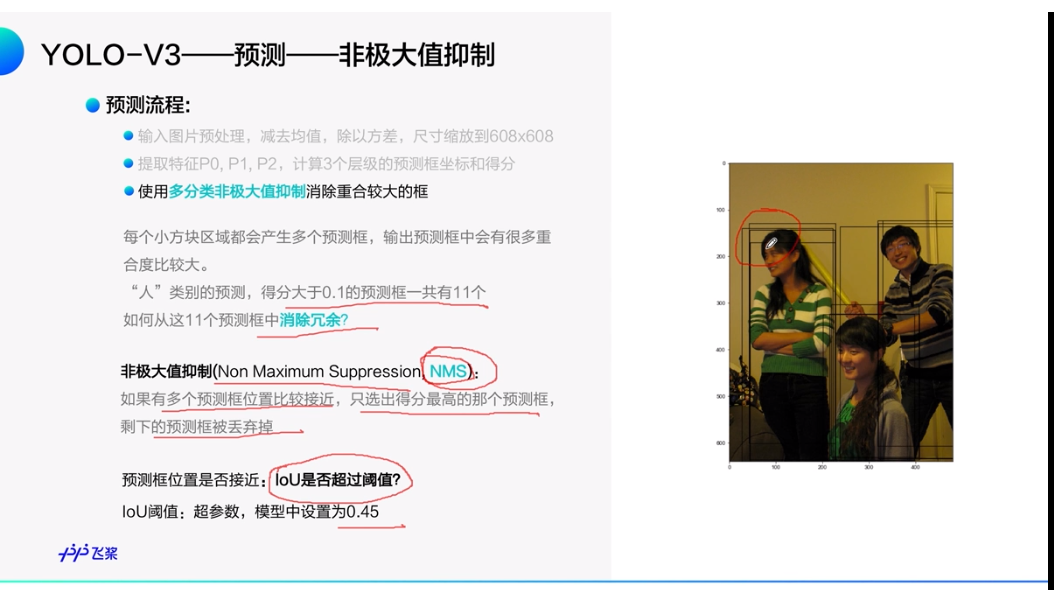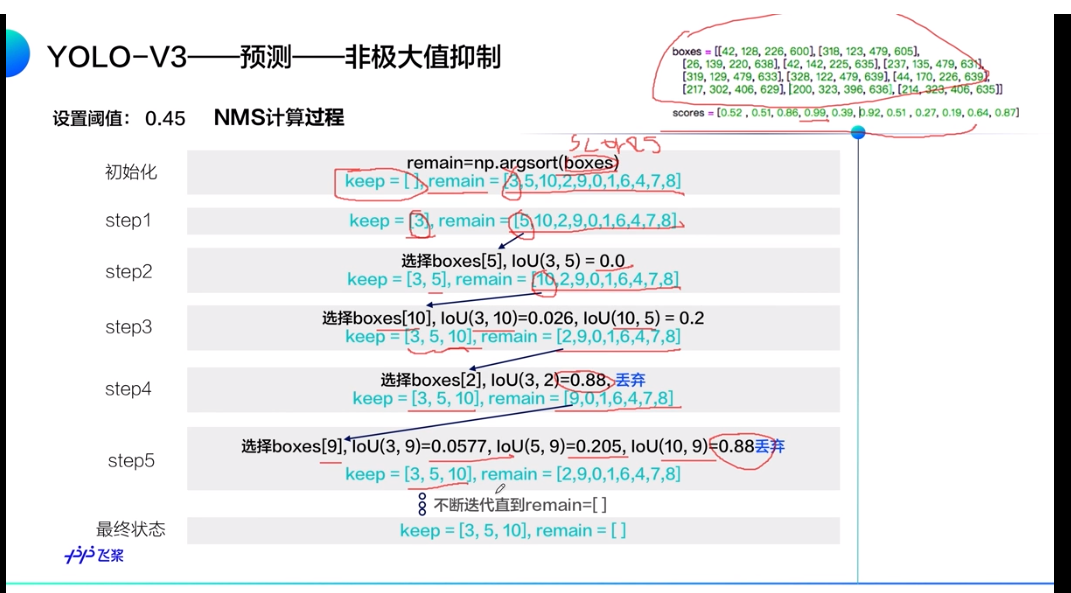上图中NMS的计算流程：右上角列出了预测框和每个预测框的分数（boxes和scores）。就是定义一个列表keep存放每次选出的分数最大且阈值小（阈值小代表两个预测框没重合，也就是框的不是一个对象）的框。step3中选择boxes，boxes要和之前keep中存在的3,5进行iou比较，如果与他俩比较的iou都比较小，说明boxes框中的对象和boxesboxes框中的对象不是同一个对象，所以保留下来。图是NMS后的结果：keep中只有三个框了。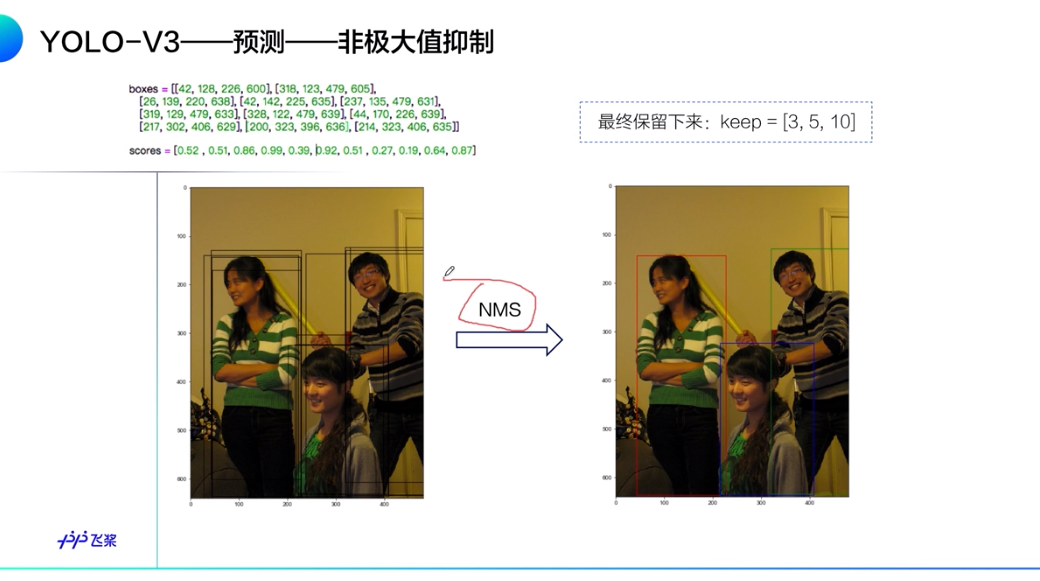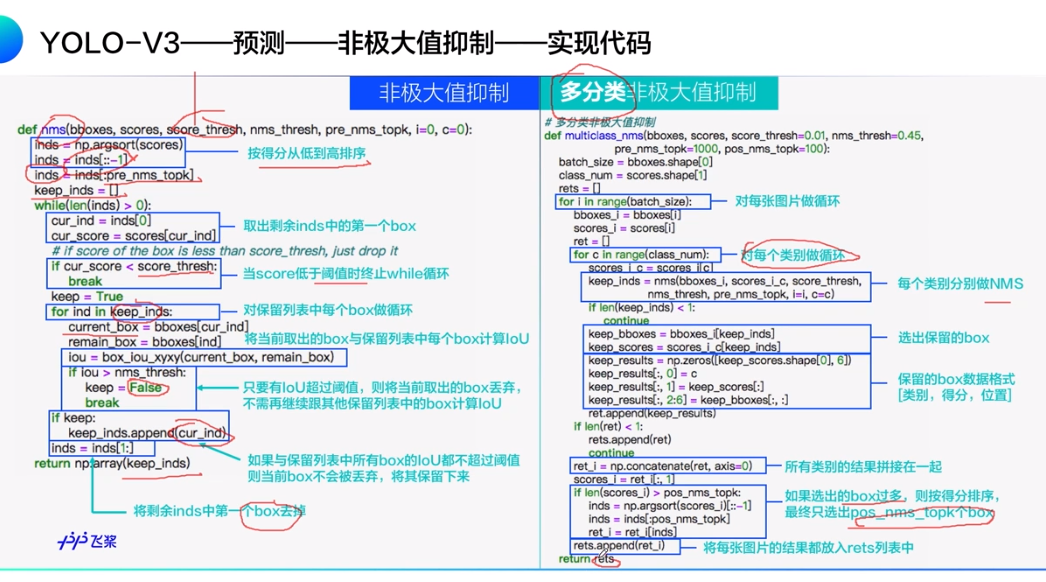展开全文深度学习
• 类似统计数据为0,1,1,2,3,2,1,1,0，0,2,3,3,5,3,5,3,0…等...//由于可能存在相同极值，所以对直方图数据进行平滑，权重为1 const double filter = {0.0536,0.1232,0.2032,0.2400,0.2032,0.1232,0.0536}; std::v
• 定义在集Ｅ子集Ｘ上两个实函数比值的极大极小值分别记为Ｍ（Ｘ）ｍ（Ｘ），极差△（Ｘ）＝Ｍ（Ｘ）－ｍ（Ｘ）。本文给出在秩为ｒ独立系统（Ｅ，Ｉ）（ＩＰ（Ｅ））中求ｍａｘ｛ｍ（Ｘ）｜Ｘ∈Ｉ｜，｜Ｘ...
• 第4章-23 求矩阵的局部极大值分析改进题目解法 分析 定义一个判断部分最大值的函数：大于四周的函数，就返回True 对非边界值的判断:范围是range(1,n-1) Python中appendextend的区别 list.append(object) 向列表...
• 由于广义半无限极大极小问题的极大函数约束集合随x变化而变化，增加了对该问题理论分析求解难度。为了克服这种情况，许多研究者考虑通过转化消除约束集合中约束f(x,y)。本文是通过一类由1范数定义的精确罚...
• 我在Logistic Regression回归中对损失函数用极大似然估计推导，在线性回归中对损失函数用最小二乘法推导，发现在推导梯度过程中，结果是一样，所以我对两种方法进行了分析对比。 一、最小二乘法 1.定义 当从模型...
• 已给m个定义在n维欧几里德空间函数,在这m个函数中求r个最大函数和的最小值,其中1≤r≤ m。这个问题在定位分析领域有重要应用。显然该问题是非光滑最优化问题,不能直接用牛顿法或拟牛顿法来求解。该问题转化为...
• 在已给q个定义于n维欧几里德空间函数中求r个最大函数和的最小值,其中1≤r≤q。该问题是非光滑最优化问题,不能直接用一阶最优化方法或梯度法求解。利用对偶理论将该问题转化为只包含最大函数max{0,t}非光滑...
• 人们定义IoU这个概念，是为了评价你对象定位算法是否精准，但更一般地说，IoU衡量了两个边界框重叠相对大小。 如果实际边界框是红色，我们算法给出这个紫色边界框，那么这个结果是好还是坏? 交并比(IoU)...吴恩达
• 基于Alpha-Beta剪枝极大极小博弈算法五子棋AI实现 ...极小极大值算法学习及应用 Alpha-Beta剪枝算法学习及应用 2、实训模块 1.棋盘绘制 绘制五子棋棋盘 2.五子棋人人对弈实现 实现双方手动下五子棋，定义下棋...算法 python twitter
• 极大极小策略 MAXMIN strategies 在零博弈背景下特别有意义。实际上对所有博弈都会很有意义。...极大极小值或安全水平，就是极大极小策略保证回报 the maxmin strategy, is a strategy that maximizes m...
• 根据函数风险规避系数的定义,说明经非线性变换后的函数满足带有风险规避系数的HJB偏微分方程。当风险规避系数无限时,给出了证券投资最优策略。最后给出一个算例,表明带有规避系数的HJB偏微分方程可得函数...
• 函数极值和最大值最小值是几个经常要求特殊点 1.在这一章节里面需要掌握有三个定理： 求解极值步骤： ...2.求解最大值和最小值问题 ...上面主要是三个定理应用判定极大值和极小值，求出极大值，..
• 进程线程提出极大的提高了操作提供性能。进程让操作系统并发性成为了可能，而线程让进程内部并发成为了可能。 多进程方式也可以实现并发，为什么我们要使用多线程？ 多进程方式确实可以实现并发，但使用...java 经验分享
• 引入了赋值域是格时离散事件系统形式化定义,并提出了由它所生成语言可控性概念,以及给出并证明了格离散事件系统可控...最后得到了给定语言的极大可控子语言和极小可控超语言存在性证明以及它们表达式。
• 在二值化图象的时候把大于某个临界灰度值的像素灰度设为灰度极大值，把小于这个值的像素灰度设为灰度极小值，从而实现二值化。 根据阈值选取的不同，二值化的算法分为固定阈值自适应阈值。 比较常用的二值化方法...
• 根据保护失效边界模型计算出保护失效边界电阻值和相应故障距离，用以绘制保护失效边界电阻曲线，再引入保护失效系数概念，以此评估该继电器耐受过渡电阻能力以及抗风险能力。PSCAD仿真结果表明，该模型可准确...
• 凹函数与凸函数相似，凸函数具有全局极小值，凹函数具有全局极大值。 因为两者很方便进行转换，我们以凸函数为例作介绍。 1. 凸函数的定义 要定义凸函数，首先必须要对凸集有所了解。 凸集： 给定集合以及其中的任意...
• D、A两点之间H称为矫顽力，即将磁芯中剩余磁性减小到零所需要磁场强度。 　图 磁滞回线 　磁导率定义为比值s／H，可从BH曲线斜率得到。当处理小的交流信号时，我们所关心区域被限制在一个相当窄...
• 1.2.1 代数体函数的定义 6 1.2.2 正则函数元素 6 1.3 函数元素的开拓 .10 1.3.1 直接开拓 10 1.3.2 解析开拓 11 1.4 Riemann 曲面 . 15 1.4.1 代数体函数决定的Riemann 曲面 . 15 1.4.2 奇异元素 17 1.5 零点与极点 ...
• hdr”头文件，这将极大地帮助客户）。 作为一家专业公司，Bruker有义务动机来帮助用户访问其数据。 该项目自1999年以来就存在变体，但情况并没有得到改善。 任何考虑从该公司购买设备或服务客户都应要求他们开发...
• ## 极值和最值的区别

千次阅读 2020-02-11 22:52:44
区别 极值 最值 定义 f(x)在U(x0)有定义，x∈U(x0)...极大值和极小值统称为极值，极大值点和极小值点统称为极值点。 f(x)在区间I有定义，x0∈I； f(x)≥f(x0)，其中∀x∈I。 称f...
• 在二值化图象的时候把大于某个临界灰度值的像素灰度设为灰度极大值，把小于这个值的像素灰度设为灰度极小值，从而实现二值化。 根据阈值选取的不同，二值化的算法分为固定阈值自适应阈值。 比较常用的二值化...图像处理
• 极值点的定义：在某点的一个邻域内，该点的函数值是最大值或最小值，则该点是个极大值点或极小值点。 极值点可能是一阶导数为0的点，也可能是一阶导数不存在的点。所以求极值点的时候，找出所有一阶导数为0的点不...
• 梯度下降算法 以下内容参考 微信公众号 AI学习与实践平台 SIGAI ...至于是极大值还是极小值，要看二阶导数/Hessian矩阵，Hessian矩阵我们将在后面文章中介绍，这是由函数二阶偏导数构成矩阵。这分为下面
• 一、数组查找（用二分法：一般求局部极小值、数组部分有序） 例 一个给定的不包含相同元素的整数数组，求它的一个局部最小值（局部极小值的定义是一个值比左右相邻的（如果存在）都小的值） #复杂度O(logn) def ......